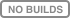# mlinsights: tricky scikit-learn¶mlinsights implements functions to get insights on machine learned models or various kind of transforms to help manipulating data in a single pipeline. It implements `QuantileLinearRegression` which trains a linear regression with L1 norm non-linear correlation based on decision trees, `QuantileMLPRegressor` which is a modification of scikit-learn’s MLPRegressor which trains a multi-layer perceptron with L1 norm…

Short example:

<<<

```from sklearn.datasets import load_boston
from sklearn.linear_model import LinearRegression
from mlinsights.mlmodel import QuantileLinearRegression

X, y = data.data, data.target

clq = QuantileLinearRegression()
clq.fit(X, y)
print(clq.coef_)

clr = LinearRegression()
clr.fit(X, y)
print(clr.coef_)
```

>>>

```    [-1.38360824e-01  3.50984183e-02  3.66268178e-02  1.34105371e+00
-1.09139098e+01  4.93325545e+00 -1.85879308e-02 -1.05912067e+00
2.13237100e-01 -1.07542971e-02 -8.08288526e-01  1.10490363e-02
-3.67998974e-01]
[-1.08011358e-01  4.64204584e-02  2.05586264e-02  2.68673382e+00
-1.77666112e+01  3.80986521e+00  6.92224640e-04 -1.47556685e+00
3.06049479e-01 -1.23345939e-02 -9.52747232e-01  9.31168327e-03
-5.24758378e-01]
```

This documentation was generated with scikit-learn version…

<<<

```from sklearn import __version__
print(__version__)
```

>>>

```    0.23.2
```